• 分类roc曲线
2021-02-03 20:33:41

from sklearn.metrics import confusion_matrix, roc_curve, auc

from sklearn.preprocessing import label_binarize

import numpy as np

y_test_bi = label_binarize(y_test, classes=[0,1,2,3,4,5,6,7,8,9,10,11,12,13,14,15,16,17,18, 19,20,21,2,23,24,25,26,27,28,29,30,31,32,33,34,35,36,37,38,3,40,41,42,43,44,45])

y_pred_bi = label_binarize(y_pred, classes=[0,1,2,3,4,5,6,7,8,9,10,11,12,13,14,15,16,17,18, 19,20,21,2,23,24,25,26,27,28,29,30,31,32,33,34,35,36,37,38,3,40,41,42,43,44,45])

# Compute ROC curve and ROC area for each class

fpr = dict()

tpr = dict()

roc_auc = dict()

for i in range(2):

fpr[i], tpr[i], _ = roc_curve(y_test_bi, y_pred_bi)

roc_auc[i] = auc(fpr[i], tpr[i])

y_test_bi和{}的形状都是{}，因为有46个类和300个测试数据点。在

这两个矩阵的格式是每列代表一个类，并且由0s或{}s组成

但我得到了一个错误：

^{pr2}\$

更多相关内容
• 基本概念 precision：预测为对的当中，原本为对的比例（越大越好，1为理想状态） recall：原本为对的当中，预测为对的比例（越大越好，1为理想状态） ...ROC曲线通常在Y轴上具有真阳性率，在X轴上具有假阳性率。
• ## 多分类ROC曲线

万次阅读 多人点赞 2019-02-14 11:13:38
一个分类模型(分类器)是一个将某个实例映射到一个特定类的过程.分类器的结果可以是给出该实例所属的类别，也可以给定该实例属于某个类别的概率。 首先来考虑一个两类预测问题(双分类器),其结果要么是真(p)要么是假...

### 1 基本概念

一个分类模型(分类器)是一个将某个实例映射到一个特定类的过程.分类器的结果可以是给出该实例所属的类别，也可以给定该实例属于某个类别的概率。
首先来考虑一个两类预测问题(双分类器),其结果要么是真(p)要么是假(n).在双分类器中有4类可能的输出.如果输出的预测是p而真实的结果也是p,那么这就叫做真阳性(TP);然而如果真实的结果是n,则这就叫做假阳性(FP).相反的来说,一个真阴性发生在预测结果和实际结果都为n的时候,而假阴性是当预测输出是n而实际值是p的时候，这几种情况可以用下面的矩阵来组织。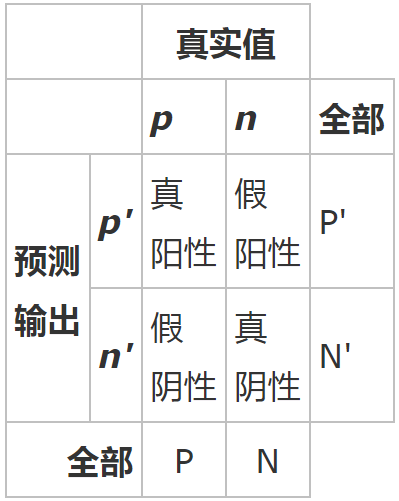举一个现实世界中的恰当的例子,考虑一个检测一个人是否得一种病的测试.一个假阳性就是一个人被测试是有这种病的,但实际却没有的情况.一个假阴性就是一个人被测试是健康的,但实际却是得病的情况。
上面那个矩阵就称为混淆矩阵：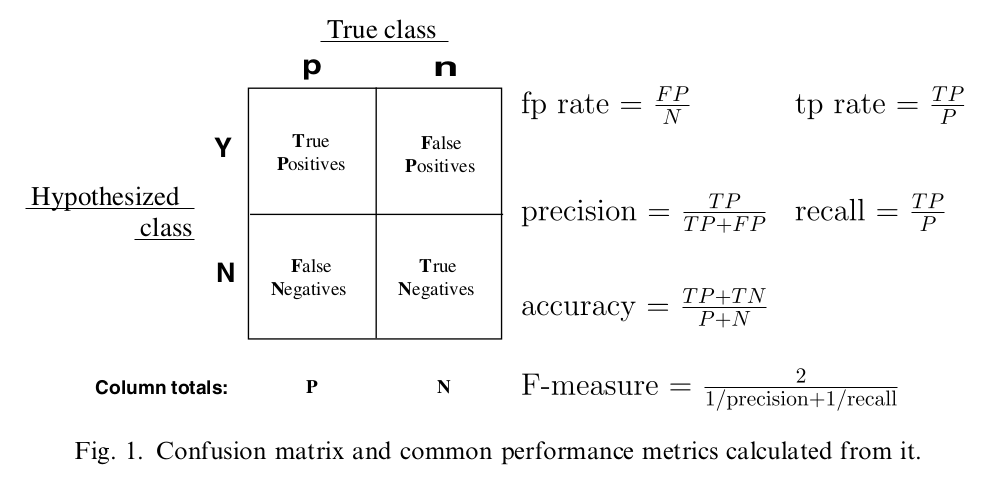由图中公式可知各个指标的含义：
precision：预测为对的当中，原本为对的比例（越大越好，1为理想状态）
recall：原本为对的当中，预测为对的比例（越大越好，1为理想状态）
F-measure：F度量是对准确率和召回率做一个权衡（越大越好，1为理想状态，此时precision为1，recall为1）
accuracy：预测对的（包括原本是对预测为对，原本是错的预测为错两种情形）占整个的比例（越大越好，1为理想状态）
fp rate：原本是错的预测为对的比例（越小越好，0为理想状态）
tp rate：原本是对的预测为对的比例（越大越好，1为理想状态）
在了解了上述的一些指标的含义以及计算公式后，接下来就可以进入ROC曲线了。
如下示例图中，曲线的横坐标为false positive rate（FPR），纵坐标为true positive rate（TPR）。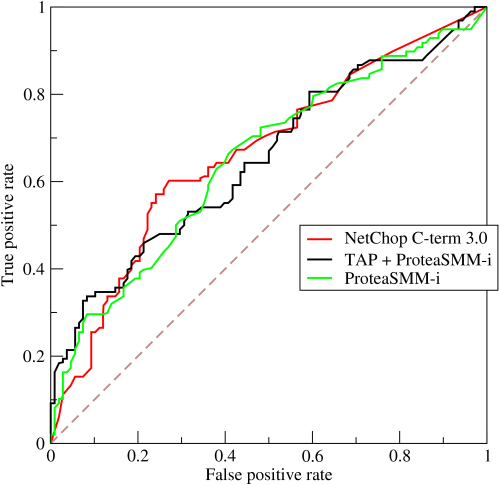要生成一个ROC曲线,只需要真阳性率(TPR)和假阳性率(FPR)。TPR决定了一个分类器或者一个诊断测试在所有阳性样本中能正确区分的阳性案例的性能.而FPR是决定了在所有阴性的样本中有多少假阳性的判断. ROC曲线中分别将FPR和TPR定义为x和y轴,这样就描述了真阳性(获利)和假阳性(成本)之间的博弈.而TPR就可以定义为灵敏度,而FPR就定义为1-特异度,因此ROC曲线有时候也叫做灵敏度和1-特异度图像.每一个预测结果在ROC曲线中以一个点代表.
有了ROC曲线后，可以引出AUC的含义：ROC曲线下的面积（越大越好，1为理想状态）

### 2 ROC曲线图中的特殊点和线

#### （1）ROC曲线图中的四个点

第一个点，(0,1)，即FPR=0, TPR=1，这意味着FN（false negative）=0，并且FP（false positive）=0。这是一个完美的分类器，它将所有的样本都正确分类。第二个点，(1,0)，即FPR=1，TPR=0，类似地分析可以发现这是一个最糟糕的分类器，因为它成功避开了所有的正确答案。第三个点，(0,0)，即FPR=TPR=0，即FP（false positive）=TP（true positive）=0，可以发现该分类器预测所有的样本都为负样本（negative）。类似的，第四个点（1,1），分类器实际上预测所有的样本都为正样本。经过以上的分析，，ROC曲线越接近左上角，该分类器的性能越好。

#### （2）ROC曲线图中的一条特殊线

考虑ROC曲线图中的虚线y=x上的点。这条对角线上的点其实表示的是一个采用随机猜测策略的分类器的结果，例如(0.5,0.5)，表示该分类器随机对于一半的样本猜测其为正样本，另外一半的样本为负样本。

### 3 如何画ROC曲线

对于一个特定的分类器和测试数据集，每一个实例都会得到一个分类结果，通过统计，利用上述公式，可以得到一组FPR和TPR结果，而要得到一个曲线，实际上需要一系列FPR和TPR的值。那么这一系列值是怎么构造出来的呢？
在ROC曲线的定义中，有“as its discrimination threshold is varied.”这样一句话，而在本文最开始也提到了分类器的结果可以是“概率输出”，即表示分类器认为某个样本具有多大的概率属于正样本（或负样本），于是如果设置不同的threshold，那么分类结果就会有所变动，因此可以得到一系列FPR和TPR的值。
接下来就是利用python实现ROC曲线，sklearn.metrics有roc_curve, auc两个函数，本文主要就是通过这两个函数实现二分类和多分类的ROC曲线。

fpr, tpr, thresholds  =  roc_curve(y_test, scores) 
1

其中y_test为测试集的结果，scores为模型预测的测试集得分（注意：通过decision_function(x_test)计算scores的值）；fpr,tpr,thresholds 分别为假正率、真正率和阈值。（应该是不同阈值下的真正率和假正率）。

roc_auc =auc(fpr, tpr) 
1

其中roc_auc为计算的acu的值。

#### （1）二分类问题中的ROC曲线

本实例中的数据来源于sklearn中的鸢尾花（iris）数据，代码来源于LZ_Zack的博客，在最后参考链接里面会给出原博客的链接。

# -*- coding: utf-8 -*-

import numpy as np
import matplotlib.pyplot as plt
from sklearn import svm, datasets
from sklearn.metrics import roc_curve, auc  ###计算roc和auc
from sklearn import cross_validation

# Import some data to play with
X = iris.data
y = iris.target

##变为2分类
X, y = X[y != 2], y[y != 2]

# Add noisy features to make the problem harder
random_state = np.random.RandomState(0)
n_samples, n_features = X.shape
X = np.c_[X, random_state.randn(n_samples, 200 * n_features)]

# shuffle and split training and test sets
X_train, X_test, y_train, y_test = cross_validation.train_test_split(X, y, test_size=.3,random_state=0)

# Learn to predict each class against the other
svm = svm.SVC(kernel='linear', probability=True,random_state=random_state)

###通过decision_function()计算得到的y_score的值，用在roc_curve()函数中
y_score = svm.fit(X_train, y_train).decision_function(X_test)

# Compute ROC curve and ROC area for each class
fpr,tpr,threshold = roc_curve(y_test, y_score) ###计算真正率和假正率
roc_auc = auc(fpr,tpr) ###计算auc的值

plt.figure()
lw = 2
plt.figure(figsize=(10,10))
plt.plot(fpr, tpr, color='darkorange',
lw=lw, label='ROC curve (area = %0.2f)' % roc_auc) ###假正率为横坐标，真正率为纵坐标做曲线
plt.plot([0, 1], [0, 1], color='navy', lw=lw, linestyle='--')
plt.xlim([0.0, 1.0])
plt.ylim([0.0, 1.05])
plt.xlabel('False Positive Rate')
plt.ylabel('True Positive Rate')
plt.legend(loc="lower right")
plt.show()
1234567891011121314151617181920212223242526272829303132333435363738394041424344454647

该实例的ROC图如下所示：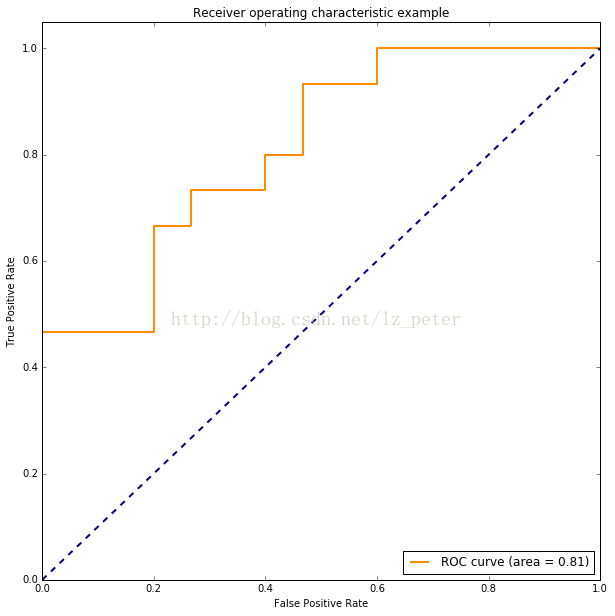#### （2）多分类问题中的ROC曲线

对于多分类问题，ROC曲线的获取主要有两种方法：
假设测试样本个数为m，类别个数为n。在训练完成后，计算出每个测试样本的在各类别下的概率或置信度，得到一个[m， n]形状的矩阵P，每一行表示一个测试样本在各类别下概率值（按类别标签排序）。相应地，将每个测试样本的标签转换为类似二进制的形式，每个位置用来标记是否属于对应的类别（也按标签排序，这样才和前面对应），由此也可以获得一个[m， n]的标签矩阵L。
①方法一：每种类别下，都可以得到m个测试样本为该类别的概率（矩阵P中的列）。所以，根据概率矩阵P和标签矩阵L中对应的每一列，可以计算出各个阈值下的假正例率（FPR）和真正例率（TPR），从而绘制出一条ROC曲线。这样总共可以绘制出n条ROC曲线。最后对n条ROC曲线取平均，即可得到最终的ROC曲线。
②方法二：
首先，对于一个测试样本：1）标签只由0和1组成，1的位置表明了它的类别（可对应二分类问题中的‘’正’’），0就表示其他类别（‘’负‘’）；2）要是分类器对该测试样本分类正确，则该样本标签中1对应的位置在概率矩阵P中的值是大于0对应的位置的概率值的。基于这两点，将标签矩阵L和概率矩阵P分别按行展开，转置后形成两列，这就得到了一个二分类的结果。所以，此方法经过计算后可以直接得到最终的ROC曲线。
上面的两个方法得到的ROC曲线是不同的，当然曲线下的面积AUC也是不一样的。 在python中，方法1和方法2分别对应sklearn.metrics.roc_auc_score函数中参数average值为’macro’和’micro’的情况。下面参考sklearn官网提供的例子，对两种方法进行实现。

# 引入必要的库
import numpy as np
import matplotlib.pyplot as plt
from itertools import cycle
from sklearn import svm, datasets
from sklearn.metrics import roc_curve, auc
from sklearn.model_selection import train_test_split
from sklearn.preprocessing import label_binarize
from sklearn.multiclass import OneVsRestClassifier
from scipy import interp

# 加载数据
X = iris.data
y = iris.target
# 将标签二值化
y = label_binarize(y, classes=[0, 1, 2])
# 设置种类
n_classes = y.shape

# 训练模型并预测
random_state = np.random.RandomState(0)
n_samples, n_features = X.shape

# shuffle and split training and test sets
X_train, X_test, y_train, y_test = train_test_split(X, y, test_size=.5,random_state=0)

# Learn to predict each class against the other
classifier = OneVsRestClassifier(svm.SVC(kernel='linear', probability=True,
random_state=random_state))
y_score = classifier.fit(X_train, y_train).decision_function(X_test)

# 计算每一类的ROC
fpr = dict()
tpr = dict()
roc_auc = dict()
for i in range(n_classes):
fpr[i], tpr[i], _ = roc_curve(y_test[:, i], y_score[:, i])
roc_auc[i] = auc(fpr[i], tpr[i])

# Compute micro-average ROC curve and ROC area（方法二）
fpr["micro"], tpr["micro"], _ = roc_curve(y_test.ravel(), y_score.ravel())
roc_auc["micro"] = auc(fpr["micro"], tpr["micro"])

# Compute macro-average ROC curve and ROC area（方法一）
# First aggregate all false positive rates
all_fpr = np.unique(np.concatenate([fpr[i] for i in range(n_classes)]))
# Then interpolate all ROC curves at this points
mean_tpr = np.zeros_like(all_fpr)
for i in range(n_classes):
mean_tpr += interp(all_fpr, fpr[i], tpr[i])
# Finally average it and compute AUC
mean_tpr /= n_classes
fpr["macro"] = all_fpr
tpr["macro"] = mean_tpr
roc_auc["macro"] = auc(fpr["macro"], tpr["macro"])

# Plot all ROC curves
lw=2
plt.figure()
plt.plot(fpr["micro"], tpr["micro"],
label='micro-average ROC curve (area = {0:0.2f})'
''.format(roc_auc["micro"]),
color='deeppink', linestyle=':', linewidth=4)

plt.plot(fpr["macro"], tpr["macro"],
label='macro-average ROC curve (area = {0:0.2f})'
''.format(roc_auc["macro"]),
color='navy', linestyle=':', linewidth=4)

colors = cycle(['aqua', 'darkorange', 'cornflowerblue'])
for i, color in zip(range(n_classes), colors):
plt.plot(fpr[i], tpr[i], color=color, lw=lw,
label='ROC curve of class {0} (area = {1:0.2f})'
''.format(i, roc_auc[i]))

plt.plot([0, 1], [0, 1], 'k--', lw=lw)
plt.xlim([0.0, 1.0])
plt.ylim([0.0, 1.05])
plt.xlabel('False Positive Rate')
plt.ylabel('True Positive Rate')
plt.title('Some extension of Receiver operating characteristic to multi-class')
plt.legend(loc="lower right")
plt.show()
123456789101112131415161718192021222324252627282930313233343536373839404142434445464748495051525354555657585960616263646566676869707172737475767778798081828384

画出的ROC曲线如下图所示。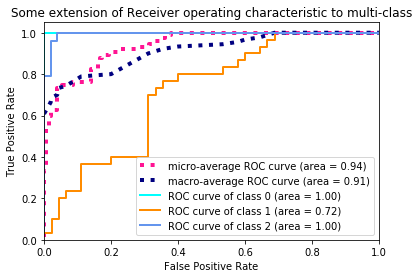如果是只需画出具体的某一类的ROC曲线，只需要修改下画图代码即可。

plt.figure()
lw = 2
plt.plot(fpr, tpr, color='darkorange',
lw=lw, label='ROC curve (area = %0.2f)' % roc_auc)
plt.plot([0, 1], [0, 1], color='navy', lw=lw, linestyle='--')
plt.xlim([0.0, 1.0])
plt.ylim([0.0, 1.05])
plt.xlabel('False Positive Rate')
plt.ylabel('True Positive Rate')
plt.legend(loc="lower right")
plt.show()
123456789101112

画出的ROC曲线如下图所示。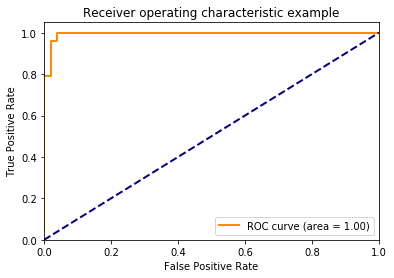### 4 参考文献

【1】(Fawcett, 2006)，Fawcett, T. (2006). An introduction to ROC analysis. Pattern recognition letters, 27(8), 861-874.
【2】Davis, J., & Goadrich, M. (2006, June). The relationship between Precision-Recall and ROC curves. In Proceedings of the 23rd international conference on Machine learning (pp. 233-240). ACM.
【3】ROC和AUC介绍以及如何计算AUC
【4】ROC曲线、AUC、Precision、Recall、F-measure理解及Python实现
【5】ROC曲线
【6】多分类下的ROC曲线和AUC
【7】用Python画ROC曲线

展开全文• 示例MATLAB脚本以加载数据并绘制 ROC 曲线并计算4种不同分类的AUC 算法： 逻辑回归的 GLM 支持向量机朴素贝叶斯分类树用法： matlab < plot_roc_curve_example_comparealgos.m 改编自...matlab
• ROC曲线，一般适用于你的分类器输出一个“概率值”，即这个样本属于某个类的概率是多少。 如此的话，你就需要设定一个阈值， 大于这个阈值属于正类，小于这个阈值属于负类。 从而，对于这个阈值P0， 就会得到对应的...
• 对于多分类问题，ROC曲线的获取主要有两种方法： 假设测试样本个数为m，类别个数为n。在训练完成后，计算出每个测试样本的在各类别下的概率或置信度，得到一个[m， n]形状的矩阵P，每一行表示一个测试样本在各类别下...

对于多分类问题，ROC曲线的获取主要有两种方法：
假设测试样本个数为m，类别个数为n。在训练完成后，计算出每个测试样本的在各类别下的概率或置信度，得到一个[m， n]形状的矩阵P，每一行表示一个测试样本在各类别下概率值（按类别标签排序）。相应地，将每个测试样本的标签转换为类似二进制的形式，每个位置用来标记是否属于对应的类别（也按标签排序，这样才和前面对应），由此也可以获得一个[m， n]的标签矩阵L。
①方法一：每种类别下，都可以得到m个测试样本为该类别的概率（矩阵P中的列）。所以，根据概率矩阵P和标签矩阵L中对应的每一列，可以计算出各个阈值下的假正例率（FPR）和真正例率（TPR），从而绘制出一条ROC曲线。这样总共可以绘制出n条ROC曲线。最后对n条ROC曲线取平均，即可得到最终的ROC曲线。
②方法二：
首先，对于一个测试样本：1）标签只由0和1组成，1的位置表明了它的类别（可对应二分类问题中的‘’正’’），0就表示其他类别（‘’负‘’）；2）要是分类器对该测试样本分类正确，则该样本标签中1对应的位置在概率矩阵P中的值是大于0对应的位置的概率值的。基于这两点，将标签矩阵L和概率矩阵P分别按行展开，转置后形成两列，这就得到了一个二分类的结果。所以，此方法经过计算后可以直接得到最终的ROC曲线。
上面的两个方法得到的ROC曲线是不同的，当然曲线下的面积AUC也是不一样的。 在python中，方法1和方法2分别对应sklearn.metrics.roc_auc_score函数中参数average值为’macro’和’micro’的情况。下面参考sklearn官网提供的例子，对两种方法进行实现。

上代码：

# # 绘制roc曲线  # #
y_test_one_hot = label_binarize(y_test_cls, np.arange(3))   # 将标签二值化
y_predict_one_hot = y_logits_cls

plt.figure()
# 绘图
mpl.rcParams['font.sans-serif'] = u'SimHei'
mpl.rcParams['axes.unicode_minus'] = False
# FPR就是横坐标,TPR就是纵坐标
# 计算ROC
fpr_dict, tpr_dict, roc_auc = dict(), dict(), dict()
for i in range(3):  # 计算每一个标签的假正例率（fpr）和真正例率（tpr）
fpr_dict[i], tpr_dict[i], _ = roc_curve(y_test_one_hot[:, i], y_predict_one_hot[:, i])
roc_auc[i] = auc(fpr_dict[i], tpr_dict[i])
# 两种画法：
# 方法一：将所有的标签进行二值化处理后，如[[0,0,1],[0,1,0]] 转成[0,0,1,0,1,0] 转成二分类进行求解
fpr_dict["micro"], tpr_dict["micro"], _ = roc_curve(y_test_one_hot.ravel(),
y_predict_one_hot.ravel())
roc_auc["micro"] = auc(fpr_dict["micro"], tpr_dict["micro"])

# # 方法二： 将每个标签的fpr和tpr进行累加除以种类数，即画出平均后的roc曲面
# n_classes = 3
# from scipy import interp
# all_fpr = np.unique(np.concatenate([fpr_dict[i] for i in range(n_classes)]))
# # Then interpolate all ROC curves at this points
# mean_tpr = np.zeros_like(all_fpr)
# for i in range(n_classes):
#     mean_tpr += interp(all_fpr, fpr_dict[i], tpr_dict[i])
# # Finally average it and compute AUC
# mean_tpr /= n_classes
# fpr_dict["macro"] = all_fpr
# tpr_dict["macro"] = mean_tpr
# roc_auc["macro"] = auc(fpr_dict["macro"], tpr_dict["macro"])
# print(roc_auc)

# 显示到当前界面，保存为svm.png
lw = 2
# plt.plot(fpr_dict, tpr_dict, color='darkorange',  # 画关于正面的roc曲面
#          lw=lw, label='ROC curve (area = %0.3f)' % roc_auc["micro"])
plt.plot(fpr_dict["micro"], tpr_dict["micro"], color='darkorange',
lw=lw, label='ROC curve (area = %0.3f)' % roc_auc["micro"])
plt.plot([0, 1], [0, 1], color='navy', lw=lw, linestyle='--')
plt.xlim([0.0, 1.0])
plt.ylim([0.0, 1.0])
plt.xlabel('False Positive Rate')
plt.ylabel('True Positive Rate')
plt.legend(loc="lower right")
plt.title(u'text_rnnROC和AUC', fontsize=17)
path = os.path.join(file_path, "img")
if not os.path.exists(path): os.makedirs(path)
plt.savefig(os.path.join(file_path, "img", "{}的ROC和AUC.png".format("model_" + str(config.model_num) + "_")))
展开全文• ## 多分类ROC曲线及AUC计算

万次阅读 多人点赞 2020-06-04 01:47:57
上一篇博客（https://blog.csdn.net/u010505915/article/details/106394765）介绍了二分类下的ROC曲线及AUC计算，本文主要介绍多分类下的ROC曲线及AUC计算。 对于多分类数据，假设测试样本个数为m，分类个数为n...

上一篇博客（https://blog.csdn.net/u010505915/article/details/106394765）介绍了二分类下的ROC曲线及AUC计算，本文主要介绍多分类下的ROC曲线及AUC计算。

对于多分类数据，假设测试样本个数为m，分类个数为n（分类label分别为：1，2，...，n），可以将这些样本及对应的label表示为矩阵形式，每行一个样本，每列为该样本是否属于该分类，从而形成一个[m, n]的标签矩阵：

 ID 分类1 分类2 分类3 A 1 0 0 B 0 0 1 C 0 1 0 D 0 0 1

模型预测完成后，会计算出测试样本在各个分类下的概率，模型预测得分也能用一个[m, n]的矩阵表示，每一行表示样本在各个类别下的概率，记该矩阵为P。

 ID 分类1 分类2 分类3 A 0.8 0.15 0.05 B 0.2 0.3 0.5 C 0.3 0.6 0.1 D 0.2 0.1 0.7

多分类下画ROC曲线有两种方法：

方法1：对每种类别，都可以从矩阵P中得到m个测试样本在该分类下的打分（矩阵P中的列），从矩阵L中获取样本的类别，从而形成一个类似二分类的得分矩阵，以上图为例，分类1 的矩阵为：

 ID label score A 1 0.8 B 0 0.2 C 0 0.3 D 0 0.2

按二分类中画ROC曲线的方法，根据以上矩阵，可以计算出各个阈值下的假正例率（FPR）和真正例率（TPR），从而画出一条ROC曲线。

对每一个类别，可以画出一条ROC曲线，总共可以画出n条ROC曲线。最后对这n条ROC曲线取平均值，即可得到最终的ROC曲线。

在python中，该方法对于sklearn里sklearn.metrics.roc_auc_score函数中参数average值为'macro'的情况。

方法2：对于一个测试样本：

（1）标签由0和1组成，1的位置表明了它的类别，0表示其他类别。1对应二分类问题中的“正”，0对应“负”

（2）如果模型对该样本分类正确，则该样本在标签矩阵L中1对应的位置在概率矩阵P中的值，即为模型预测该分类的概率值。

基于以上两点，将标签矩阵L和概率矩阵P分别按行展开（即sklearn里的ravel()函数），然后转置形成两列，这就得到了一个二分类结果，根据结果直接画出ROC曲线。

在python中，该方法对于sklearn里sklearn.metrics.roc_auc_score函数中参数average值为'micro'的情况。

代码示例：

#-*-coding:utf-8-*-

import numpy as np
import matplotlib.pyplot as plt
from itertools import cycle
from sklearn.metrics import roc_curve, auc
from scipy import interp

y_label = np.array([
[1, 0, 0], [1, 0, 0], [1, 0, 0],
[0, 1, 0], [0,  1, 0], [0, 1, 0],
[0, 0, 1], [0, 0, 1], [0, 0, 1]
])

y_score = np.array([
[0.8, 0.1, 0.1], [0.2, 0.32, 0.48], [0.6, 0.1, 0.3],
[0.2, 0.5, 0.3], [0.1, 0.6, 0.3], [0.2, 0.75, 0.05],
[0.05, 0.05, 0.9], [0.1, 0.3, 0.6], [0.12, 0.8, 0.08],
])

n_classes = 3

# 计算每一类的ROC
fpr = dict()
tpr = dict()
roc_auc = dict()
for i in range(n_classes):
fpr[i], tpr[i], _ = roc_curve(y_label[:, i], y_score[:, i])
roc_auc[i] = auc(fpr[i], tpr[i])

# micro（方法二）
fpr["micro"], tpr["micro"], _ = roc_curve(y_label.ravel(), y_score.ravel())
roc_auc["micro"] = auc(fpr["micro"], tpr["micro"])

# macro（方法一）
# First aggregate all false positive rates
all_fpr = np.unique(np.concatenate([fpr[i] for i in range(n_classes)]))
# Then interpolate all ROC curves at this points
mean_tpr = np.zeros_like(all_fpr)
for i in range(n_classes):
mean_tpr += interp(all_fpr, fpr[i], tpr[i])
# Finally average it and compute AUC
mean_tpr /= n_classes
fpr["macro"] = all_fpr
tpr["macro"] = mean_tpr
roc_auc["macro"] = auc(fpr["macro"], tpr["macro"])

# Plot all ROC curves
lw=2
plt.figure()
plt.plot(fpr["micro"], tpr["micro"],
label='micro-average ROC curve (area = {0:0.2f})'
''.format(roc_auc["micro"]),
color='deeppink', linestyle=':', linewidth=4)

plt.plot(fpr["macro"], tpr["macro"],
label='macro-average ROC curve (area = {0:0.2f})'
''.format(roc_auc["macro"]),
color='navy', linestyle=':', linewidth=4)

colors = cycle(['aqua', 'darkorange', 'cornflowerblue'])
for i, color in zip(range(n_classes), colors):
plt.plot(fpr[i], tpr[i], color=color, lw=lw,
label='ROC curve of class {0} (area = {1:0.2f})'
''.format(i, roc_auc[i]))

plt.plot([0, 1], [0, 1], 'k--', lw=lw)
plt.xlim([0.0, 1.0])
plt.ylim([0.0, 1.05])
plt.xlabel('False Positive Rate')
plt.ylabel('True Positive Rate')
plt.title('multi-calss ROC')
plt.legend(loc="lower right")
plt.show()

ROC曲线：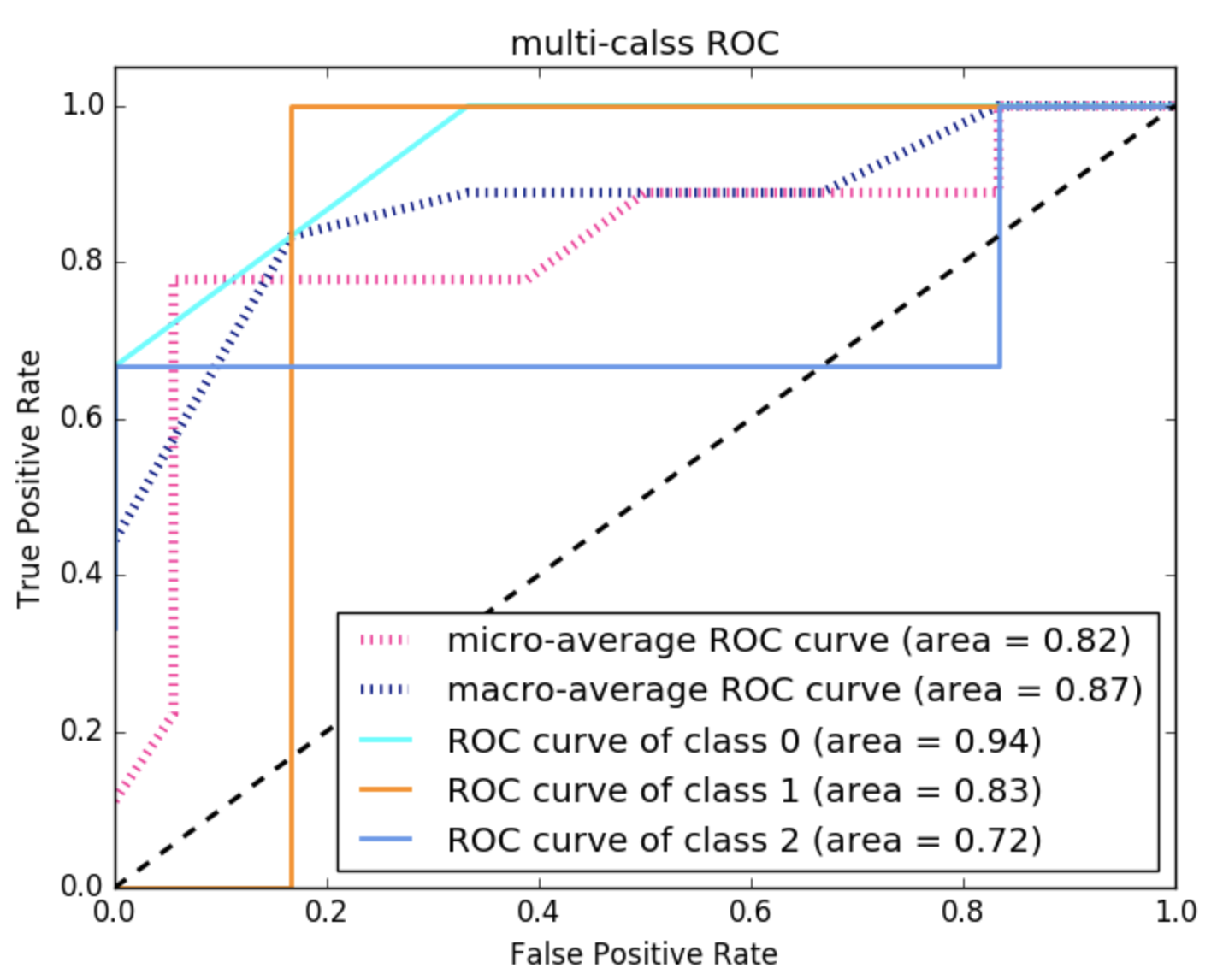参考文献：

https://blog.csdn.net/u011047955/article/details/87259052

展开全文• ROC原理介绍及利用python实现二分类和多分类ROC曲线 注：宏平均指n条ROC曲线的平均。根据all_fpr（代表横坐标值，所以去除重复并排序），当前类的fpr和tpr决定的当前类的ROC曲线，利用interp函数对fpr中相对all_...
• LDC KNN SVM QDC 测试brest-cancer数据，得出分类正确率和roc曲线
• 2分类.png") plt.show() print("--- %s seconds ---" % (time.time() - start_time)) ROC图如下所示： 多分类问题：ROC曲线 ROC曲线通常用于二分类以研究分类器的输出。为了将ROC曲线和ROC区域扩展到多类或多标签...python keras
• 答案是可以利用roc曲线来确定比较好的划分阈值。 ROC曲线介绍 二分类过程，设定阈值，大于该分数为1，小于该分数为0，统计计算TP， FN， FP，TN等数据计算FPR，TPR p(positive): 标签1 n(negative): 标签0 t(true)...阈值
• I want to draw ROC curves for each of my 46 classes. I have 300 test samples for which I've run my classifier to make a prediction.y_test is the true classes, and y_pred is what my classifier predicte...
• 主要介绍了基于python实现ROC曲线绘制广场解析,文中通过示例代码介绍的非常详细，对大家的学习或者工作具有一定的参考学习价值,需要的朋友可以参考下
• 通过ROC曲线能够有效评估算法的性能，默认情况下适用于二分类任务，在多分类任务中利用one vs rest方式计算各个类别的混淆矩阵，使用如下平均方式 macro：分别求出每个类，再进行算术平均 优点：直观、易懂，并且...sklearn
• 分类器性能指标之ROC曲线、AUC值一roc曲线1、roc曲线：接收者操作特征(receiveroperating characteristic),roc曲线上每个点反映着对同一信号刺激的感受性。横轴：负正类率(false postive rate FPR)特异度，划分实例...
• 分类问题的ROC曲线绘制 目录 多分类问题的ROC曲线绘制 #roc曲线 （1）ROC曲线图中的四个点 （2）ROC曲线图中的一条特殊线 #ROC指标 #多分类ROC曲线绘制 #roc曲线 （1）ROC曲线图中的四个点 ...sklearn python 机器学习 人工智能 数据挖掘
• 本文通过对这些指标的原理做一个简单的介绍，然后用python分别实现二分类和多分类ROC曲线。 1 基本概念 一个分类模型(分类器)是一个将某个实例映射到一个特定类的过程.分类器的结果可以是给出该实例所属的...python 机器学习
• 今天小编就为大家分享一篇浅谈ROC曲线的最佳阈值如何选取，具有很好的参考价值，希望对大家有所帮助。一起跟随小编过来看看吧
• ## ROC 二分类曲线

千次阅读 2019-10-31 17:53:57
由于工作涉及到了ROC曲线，自己不是很懂，就在网上找了资料自学了一下。 明白每一行代码，甚至可以改代码的时候，应该就学得差不多了。 这次我选用了著名的iris鸢尾花数据集作为数据源。（R,python，学习经常用到） ...python
• 给大家介绍了如何利用Python画ROC曲线，以及AUC值的计算，有需要的朋友们可以参考借鉴，下面来一起看看吧。
• from rasa_nlu.model import Interpreter from sklearn.metrics import precision_score from sklearn.metrics import recall_...print(metrics.roc_auc_score(label_one_hot,arrays,average='weighted'))sklearn
• 文章目录一、为什么采用ROC作为分类性能指标二、ROC1、roc曲线2、混淆矩阵3、绘制roc曲线三、二分类ROC曲线绘制四、多分类ROC曲线绘制参考文献 一、为什么采用ROC作为分类性能指标   既然已经这么多标准，为什么...学习笔记
• ## ROC曲线绘制(python+sklearn+多分类)

千次阅读 热门讨论 2020-07-29 13:06:51sklearn python
• 使用multiROC包可实现多分类ROC曲线的绘制 require(multiROC) data(iris) head(iris) set.seed(123456) total_number (iris) #分割数据集 train_idx (total_number, round(total_number*0.6)) train_df [train_idx, ...r语言
• 本节主要是通过著名的莺尾花数据来介绍如何画出ROC曲线。Iris 鸢尾花数据集是一个经典数据集，在统计学习和机器学习领域都经常被用作示例。数据集内包含 3 类共 150 条记录，每类各 50 个数据，每条记录都有 4 项...机器学习
• labelme的json文件转YOLO的xml文件凯哥英语视频前言数据文件下载效果图代码解释一共5个分类 , [0,1,2,3,4] , 对应的准确率为0 , 1 , 2, 3 , 4 的列代码部分 前言 基础好的就别看了 , 我用的死方法 , 数据转化都不会...python...